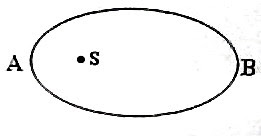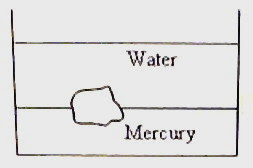## Pages

`“Life is like riding a bicycle.  To keep your balance you must keep moving.”–Albert Einstein`

## Tuesday, July 14, 2009

### AP Physics B & C – Multiple Choice Questions (MCQ) for Practice on Gravitation

Equations to be remembered in respect of the section on gravitation were given in the post dated 9th May 2008. A few multiple choice practice questions were discussed in the post dated 12th May 2008 followed by a free response practice question on gravitation in the post dated 15th May 2008. You can access all posts on gravitation on this site by clicking on the label ‘gravitation’ below this post.

Today we will discuss a few more multiple choice practice questions on gravitation. The following questions are meant for AP Physics B as well as C:

(1) The speed of an artificial satellite moving in an orbit of radius r around the earth is increased by 41.4%. The satellite will

(a) continue to move in the same orbit

(b) move in an orbit of radius 1.414 r

(c) move in an orbit of radius 0.414 r

(d) move in an orbit of radius 0.586 r

(e) escape into the outer space

The orbital speed v of any satellite is given by

v =√(gr) where g is the acceleration due to gravity at the orbit of radius r.

[You will get this by equating the centripetal force to the gravitational pull:

mv2/r = mg]

The escape velocity (vescape) of a body at a point distant r from the centre of the earth where the acceleration due to gravity is equal to g is given by

vescape =√(2gr)

Thus the escape velocity is √2 times the orbital speed.

The speed v of the satellite becomes 1.414 times the initial value on increasing the speed by 41.4%. Now, 1.414 v = √2 v. Therefore the new speed is the escape speed so that the satellite will escape into the outer space.

(2) A launch vehicle carrying an artificial satellite of mass m is set for launch on the surface of the earth of mass M and radius R. What is the minimum energy to be spent by the launch vehicle on the satellite so that the satellite will move in a circular orbit of radius 7R? (Gravitational constant = G)

(a) GMm /7R

(b) 6GMm /7R

(c) 6GMm /14R

(d) 13GMm /14R

(e) GMm /14R

The satellite of mass m is initially at rest and therefore its initial energy (Ei) is its gravitational potential energy given by

Ei = – GMm/R

When the satellite is in its orbit of radius 7R, its final energy (Ef) is given by

Ef = – GMm/(2×7R) = – GMm/(14R)

[Note that the total energy of a satellite in an orbit of radius r is – GMm/2r]

The minimum energy required for placing the satellite in its orbit is Ef Ei given by

Ef Ei = – [GMm/(14R)] – [– GMm/R]

= (GMm/R) – [GMm/(14R)]

= 13GMm /14R

(3) A small body is projected vertically up with a speed equal to half the escape speed from the earth’s surface. If the radius of the earth is R, what is the maximum height (h) reached by the body? (Neglect air resistance).

(a) R/3

(b) R/2

(c) R

(d) 2R

(e) 5R

When the body projected vertically reaches the maximum height, its speed will be zero since its initial kinetic energy us used up in increasing its gravitational potential energy. Therefore we have

½ m (ve/2)2 = [–GMm /(R+h)] – [–GMm /R] where m is the mass of the body, M is the mass of the earth, ve is the escape speed from the earth’s surface and G is the gravitational constant.

[The first term on the right hand side of the above equation is the gravitational potential energy of the body at height h. The second term is the gravitational potential energy of the body on the surface of the earth].

Since ve = √(2GM/R), the above equation becomes

GM /4R = (GM /R) –[GM /(R+h)]

Or, GM /4R = GMh /[R(R+h)

Therefore, ¼ = h/(R+h) from which h = R/3(4) The orbit of a planet moving around the sun is elliptical with the sun S at one focus of the ellipse. If the orbital speeds of the planet while at points A and B (Fig.) are V1 and V2 and the distances SA and SB are d1 and d2 respectively, V1/ V2 is equal to

(a) √(d1 /d2)

(b) √(d2 /d1)

(c) d1 /d2

(d) d2 /d1

(e) (d2 + d1) /(d2 d1)To obtain the answer you may apply Kepler’s law of areas (in respect of plnetary motion), which says that the straight line joining the sun to the planet sweeps equal areas in equal intervals of time. The adjoining figure shows path lengths CD and EF traced by the planet in equal intervals (say, ∆t) and hence the areas CADS and EBFS must be equal. If ∆t is sufficiently small, CD and EF will become straight line segments and the areas will become triangular. Equating the areas of these triangles, we have

½ ×CD×AS = ½ ×EF×BS

Or, ½ × V1 ∆t × d1 = ½ × V2 ∆t × d2

Therefore, V1/ V2 = d2 /d1

[You can easily get the answer from angular momentum conservation:

m V1 d1 = m V2 d2

where m is the mass of the planet. This gives V1/ V2 = d2 /d1]

(5) We know that the gravitational force between two point masses is inversely proportional to the square of the distance between them. If the gravitational force between two point masses were (let us imagine) inversely proportional to the nth power of the distance between them, what would be the relation between the orbital period (T) of a planet around the sun and the mean distance (r) of the planet from the sun?

(a) T2 α r2n

(b) T2 α rn

(c) T2 α r2n+1

(d) T2 α r(n+1)

(e) T2 α r(n – 1)

For simplicity, let us assume that the orbit is circular. (Circular orbit is only a special case of the general elliptical orbit and the relation between T and r will be unchanged). The centripetal force required for the circular motion is supplied by the gravitational pull so that we have

mrω2 = GMm/rn where m is the mass of the planet, ω is its orbital angular velocity, M is the mass of the sun and G is the gravitational constant.

The above equation gives ω2 = GM/r(n+1)

Since ω = T/2π, we have

(T/2π)2 = GM/r(n+1)

Therefore, T2 α r(n+1)

You will find some useful multiple choice questions on gravitation (with solution) at physicsplus.

## Sunday, July 5, 2009

### AP Physics B - Multiple Choice Questions on Fluid Mechanics

Essential things you need to remember in fluid mechanics were discussed in the post dated 3rd December 2007. A few multiple choice questions (with solution) in this section were discussed in the posts dated 4th and 5th December 2007. You can access all posts related to fluid mechanics on this site by clicking on the label ‘fluid mechanics’ below this post.

Today we will discuss a few more multiple choice questions on fluid mechanics:

(1) A water storage tank has a square hole of side 2 cm at its bottom. A plumber, unaware of the hole, admits water into the tank at a constant rate of √2 litre per second. Up to what height can water remain in the tank? (g = 10 ms–2)

(a) 12.25 m

(b) 8.25 m

(c) 2.45 m

(d) 1.25 m

(e) 0.625 m

If h is the height up to which water can remain in the tank, the velocity v of water flowing out through the hole in the steady state is given by

v = √(2gh)

The volume of water flowing out in the steady state is av where a is the area of the hole.

This must be equal to the volume of incoming water which is given as √2 litre = √2 ×10–3

m3. Thus we have

a√(2gh) = √2 ×10–3

Here a = 4 cm2 = 4×10–4 m2.

Substituting, 4×10–4×√(2×10×h) = √2 ×10–3

Or, √(10h) = 2.5 from which h = 6.25/10 = 0.625 m.(2) A piece of granite floats at the interface of mercury and water contained in a beaker (Fig.). If the densities of granite, water and mercury are ρ, ρ1 and ρ2 respectively, the ratio of the volume of granite in water to the volume in mercury is

(a) (ρ2 ρ) /(ρ ρ1)

(b) (ρ2 + ρ) /(ρ+ ρ1)

(c) ρ1 ρ2 /ρ

(d) ρ1 /ρ2

(e) ρ2 /ρ1

The weight of a floating body is equal to the weight of the displaced fluid. If V and v represent the total volume of the piece of granite and volume of granite in water respectively, we have

V ρg = v ρ1g + (V v) ρ2g

Or, v(ρ1 ρ2) = V(ρ ρ2)

Therefore, v/V = (ρ ρ2) /(ρ1 ρ2)

The ratio required in the question is v/ (Vv) and is given by

v/ (Vv) = (ρ ρ2) /[(ρ1 ρ2) (ρ ρ2)]

Or, v/ (Vv) = (ρ ρ2) /(ρ1 ρ) = (ρ2 ρ) /(ρ ρ1)

(3) The pressure of water at the bottom of a water tank exceeds the atmospheric pressure by 104 pascal. The velocity of efflux of water through an orifice at the bottom of the water tank will be (g = 10 ms–2)

(a) √5 ms–1

(b) √10 ms–1

(c) √15 ms–1

(d) √20 ms–1

(e) √30 ms–1

If h is the height of water column in the tank, we have

hρg = 104

Since the density (ρ) of water is 1000 kg m–3,

h×1000×10 = 104 from which h = 1m.

The velocity of efflux (Torricelli’s theorem) is √(2gh) = √(2×10×1) = √20 ms–1

(4) In a wind tunnel the flow speeds (of air) on the upper and lower surfaces of the wing of a model airplane are v1 and v2 respectively (v1> v2). If the wing area is A and the density of air is ρ, the lift on the wing is

(a) ρ (v12 v22)A

(b) ½ ρ (v1 v2)A

(c) ½ ρ (v12 v22)A

(d) ½ (v12 v22)A /ρ

(e) ρ (v1 v2)A

If P1 and P2 are the pressures on the upper and lower surfaces of the wing we have, by Bernoulli’s theorem,

P1 + ½ ρ v12 = P2 + ½ ρ v22

[We have ignored the small height difference between the top and bottom of the wing so that the gravitational potential energy is treated as constant].

The pressure at the top is less than the pressure at the bottom since v1> v2.

The lift on the wing is (P2 P1)A = ½ ρ (v12 v22)A

You will find similar useful multiple choice questions with solution here as well as here at Physicsplus.